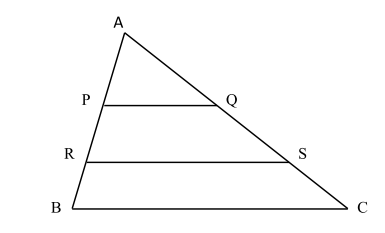# Free SAT Test Questions

Question 1 of 1
ID: SAT-AM-7
Section: Math - Additional Topics in Math
Topic: Geometry
Difficulty level: Challenging

(Practice Mode: Single selected Question » Back to Overview)

In the graphic $\Delta ABC$ given below, $PQ{\parallel}RS{\parallel}BC$ and $AP=\frac{1}{4}AB$ and $PR=\frac{1}{3}AB$. How many of the following statements are true? (Without calculator)

1. $AQ : SC$ = 3 : 5
2. $RS : BC$ = 7 : 10
3. Area of $\Delta APQ$ : Area of quadrilateral $PQSR$ = 9 : 40ANone
BAny one
CAny two
DAll three

#### Finding the Right Time to Take the SAT

Read on the optimal timings to sit for the SAT that will give you a competitive edge. With the SAT only being offered a few times every year, it is critical to find the right time to take your exam.